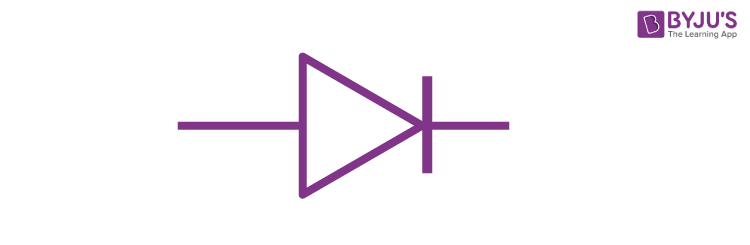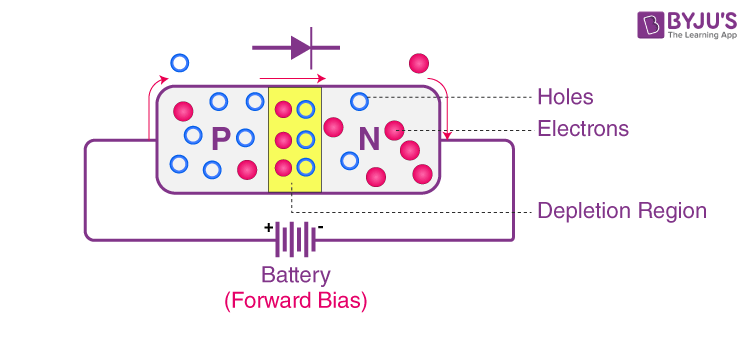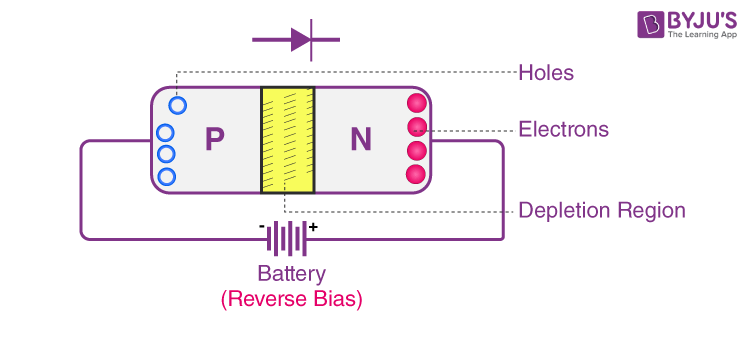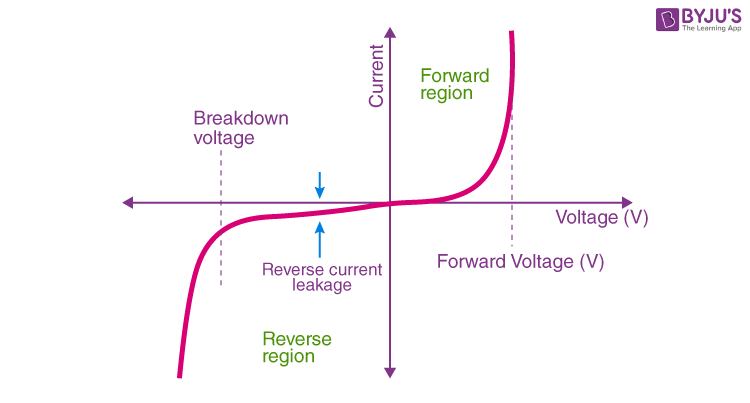# Semiconductor diode

A diode is a two-terminal electronic component that has a low resistance to the flow of current in one direction thus allowing the passage of current in one direction whereas there will be a high resistance in the other, thus restricting the flow of current in that direction. Semiconductor diodes are two-terminal devices that consist of a p-n junction and metallic contacts at their two ends.

## What is a Semiconductor Diode

A semiconductor diode is a p-n junction diode. It is a two-terminal device that conducts current only in one direction.

The figure below represents the symbol for the p-n junction diode, which symbolises the direction of the current. By applying an external voltage V we can vary the potential barrier.

## Semiconductor Diode SymbolA p-n junction is denoted by the symbol shown in the figure above. Here, the direction of the arrow indicates the permissible direction of the current.

## P-N Junction Diode Under Forward BiasWhen we apply the external voltage across the semiconductor diode in such a way that the p-side is connected to the positive terminal of the battery and the n-side is connected to the negative terminal, then the semiconductor diode is said to be forward-biased. In this case, the built-in potential of the diode and thus the width of the depletion region decreases, and the height of the barrier gets reduced. The overall barrier voltage, in this case, comes out to be V0-V, which is the difference between the built-in potential and the applied potential. As we supply a small amount of voltage, the reduction in the barrier voltage from the above-given formula is very less and thus only a small number of current carriers cross the junction in this case. Whereas, if the potential is increased by a significant value, the reduction in the barrier height will be more, thus allowing the passage of more number of carriers.

## P-N Junction Diode Under Reverse BiasWhen we apply the external voltage across the semiconductor diode in such a way that the positive terminal of the battery is connected to its n-side and the negative terminal of the battery is connected to the p-side of the diode, then it is said to be in the condition of reverse bias. When an external voltage is applied across the diode, as the direction of the external voltage is the same as that of the barrier potential, the total voltage barrier sums up to be (V0+V). Also, the width of the depletion region increases. As a result of this, the motion of carriers from one side of the junction to another decreases significantly.

## Semiconductor Diode Characteristics

The V-I characteristics curve of a semiconductor diode is given below. This characteristic curve is a typical explanation for the V-I characteristic of a semiconductor diode. Current in the semiconductor diode starts to conduct when the current exceeds the threshold of the forward voltage, which is mentioned by the manufacturer.## Types of Semiconductor Diode

Following are the types of semiconductor diodes:

• LED
• Zener diode
• Rectifier diode
• Tunnel diode
• Variable capacitance diode
• Photodiode
• Switching diode
• Gunn diode

## Applications of Semiconductor Diode

Following are the applications of semiconductor diodes:

• Rectifier diode which is used for the rectification of alternating current.
• Gunn diode which is one of the components of high-frequency electronics.
• Zener diodes are used for the stabilisation of current and voltage in electronic systems.
• Photodiode works as a photo-detector.
• Switching diode which is used for fast switching requirements.
• A tunnel diode is a special diode that is used in the negative dynamic resistance region.
• LED is used for emitting an infrared light spectrum.
• A variable capacitance diode is used when a voltage is applied in reverse biased condition.

## Frequently Asked Questions – FAQs

### What is a semiconductor diode?

A semiconductor diode is a two-terminal p-n junction diode that conducts current only in one direction.

### What are the types of semiconductor diodes?

The following are the types of light interference:

• LED
• Zener diode
• Rectifier diode
• Tunnel diode
• Variable capacitance diode
• Photodiode
• Switching diode
• Gunn diode

### Which type of the diode is used for the rectification of alternating current?

Rectifier diode is used for the rectification of alternating current.

TRUE

### What is the zero bias condition in the p-n junction diode?

A zero bias condition in the p-n junction diode is seen when no external voltage is applied to the P-N junction diode.

Stay tuned to BYJU’S and Fall in Love with Learning!

Test your knowledge on Semiconductor Diode